Open In App

# Creating a PySpark DataFrame

In this article, we will learn how to create a PySpark DataFrame. PySpark applications start with initializing SparkSession which is the entry point of PySpark as shown below.

# SparkSession initialization

from pyspark.sql import SparkSession

spark = SparkSession.builder.getOrCreate()

Note: PySpark shell via pyspark executable, automatically creates the session within the variable spark for users. So you’ll also run this using shell.

## Creating a PySpark DataFrame

A PySpark DataFrame are often created via pyspark.sql.SparkSession.createDataFrame. There are methods by which we will create the PySpark DataFrame via pyspark.sql.SparkSession.createDataFrame. The pyspark.sql.SparkSession.createDataFrame takes the schema argument to specify the schema of the DataFrame. When it’s omitted, PySpark infers the corresponding schema by taking a sample from the data.

Syntax

pyspark.sql.SparkSession.createDataFrame()

Parameters:

• dataRDD: An RDD of any kind of SQL data representation(e.g. Row, tuple, int, boolean, etc.), or list, or pandas.DataFrame.
• schema: A datatype string or a list of column names, default is None.
• samplingRatio: The sample ratio of rows used for inferring
• verifySchema: Verify data types of every row against schema. Enabled by default.

Returns: Dataframe

Below there are different ways how are you able to create the PySpark DataFrame:

### Create PySpark DataFrame from an inventory of rows

In the given implementation, we will create pyspark dataframe using an inventory of rows. For this, we are providing the values to each variable (feature) in each row and added to the dataframe object. After doing this, we will show the dataframe as well as the schema.

## Python3

 `# Need to import to use date time``from` `datetime ``import` `datetime, date` `# need to import for working with pandas``import` `pandas as pd` `# need to import to use pyspark``from` `pyspark.sql ``import` `Row` `# need to import for session creation``from` `pyspark.sql ``import` `SparkSession` `# creating the session``spark ``=` `SparkSession.builder.getOrCreate()` `# schema creation by passing list``df ``=` `spark.createDataFrame([``    ``Row(a``=``1``, b``=``4.``, c``=``'GFG1'``, d``=``date(``2000``, ``8``, ``1``),``        ``e``=``datetime(``2000``, ``8``, ``1``, ``12``, ``0``)),``  ` `    ``Row(a``=``2``, b``=``8.``, c``=``'GFG2'``, d``=``date(``2000``, ``6``, ``2``),``        ``e``=``datetime(``2000``, ``6``, ``2``, ``12``, ``0``)),``  ` `    ``Row(a``=``4``, b``=``5.``, c``=``'GFG3'``, d``=``date(``2000``, ``5``, ``3``),``        ``e``=``datetime(``2000``, ``5``, ``3``, ``12``, ``0``))``])` `# show table``df.show()` `# show schema``df.printSchema()`

Output: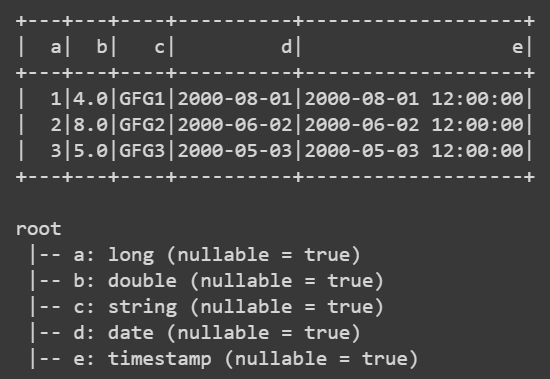### Create PySpark DataFrame with an explicit schema

In the given implementation, we will create pyspark dataframe using an explicit schema. For this, we are providing the feature values in each row and added them to the dataframe object with the schema of variables(features). After doing this, we will show the dataframe as well as the schema.

## Python3

 `# Need to import to use date time``from` `datetime ``import` `datetime, date` `# need to import for working with pandas``import` `pandas as pd` `# need to import to use pyspark``from` `pyspark.sql ``import` `Row` `# need to import for session creation``from` `pyspark.sql ``import` `SparkSession` `# creating the session``spark ``=` `SparkSession.builder.getOrCreate()` `# PySpark DataFrame with Explicit Schema``df ``=` `spark.createDataFrame([``    ``(``1``, ``4.``, ``'GFG1'``, date(``2000``, ``8``, ``1``),``     ``datetime(``2000``, ``8``, ``1``, ``12``, ``0``)),``  ` `    ``(``2``, ``8.``, ``'GFG2'``, date(``2000``, ``6``, ``2``),``     ``datetime(``2000``, ``6``, ``2``, ``12``, ``0``)),``  ` `    ``(``3``, ``5.``, ``'GFG3'``, date(``2000``, ``5``, ``3``),``     ``datetime(``2000``, ``5``, ``3``, ``12``, ``0``))``], schema``=``'a long, b double, c string, d date, e timestamp'``)` `# show table``df.show()` `# show schema``df.printSchema()`

Output:### Create PySpark DataFrame from DataFrame Using Pandas

In the given implementation, we will create pyspark dataframe using Pandas Dataframe. For this, we are providing the list of values for each feature that represent the value of that column in respect of each row and added them to the dataframe. After doing this, we will show the dataframe as well as the schema.

## Python3

 `# Need to import to use date time``from` `datetime ``import` `datetime, date` `# need to import for working with pandas``import` `pandas as pd` `# need to import to use pyspark``from` `pyspark.sql ``import` `Row` `# need to import for session creation``from` `pyspark.sql ``import` `SparkSession` `# creating the session``spark ``=` `SparkSession.builder.getOrCreate()` `## PySpark DataFrame from a pandas DataFrame``pandas_df ``=` `pd.DataFrame({``    ``'a'``: [``1``, ``2``, ``3``],``  ` `    ``'b'``: [``4.``, ``8.``, ``5.``],``  ` `    ``'c'``: [``'GFG1'``, ``'GFG2'``, ``'GFG3'``],``  ` `    ``'d'``: [date(``2000``, ``8``, ``1``), date(``2000``, ``6``, ``2``),``          ``date(``2000``, ``5``, ``3``)],``  ` `    ``'e'``: [datetime(``2000``, ``8``, ``1``, ``12``, ``0``),``          ``datetime(``2000``, ``6``, ``2``, ``12``, ``0``),``          ``datetime(``2000``, ``5``, ``3``, ``12``, ``0``)]``})` `df ``=` `spark.createDataFrame(pandas_df)``df` `# show table``df.show()` `# show schema``df.printSchema()`

Output:### Create PySpark DataFrame from RDD

In the given implementation, we will create pyspark dataframe using a list of tuples. For this, we are creating the RDD by providing the feature values in each row using the parallelize() method and added them to the dataframe object with the schema of variables(features). After doing this, we will show the dataframe as well as the schema.

## Python3

 `# Need to import to use date time``from` `datetime ``import` `datetime, date` `# need to import for working with pandas``import` `pandas as pd` `# need to import to use pyspark``from` `pyspark.sql ``import` `Row` `# need to import for session creation``from` `pyspark.sql ``import` `SparkSession` `# creating the session``spark ``=` `SparkSession.builder.getOrCreate()` `# pyspark dataframe``rdd ``=` `spark.sparkContext.parallelize([``    ``(``1``, ``4.``, ``'GFG1'``, date(``2000``, ``8``, ``1``), datetime(``2000``, ``8``, ``1``, ``12``, ``0``)),``    ``(``2``, ``8.``, ``'GFG2'``, date(``2000``, ``6``, ``2``), datetime(``2000``, ``6``, ``2``, ``12``, ``0``)),``    ``(``3``, ``5.``, ``'GFG3'``, date(``2000``, ``5``, ``3``), datetime(``2000``, ``5``, ``3``, ``12``, ``0``))``])``df ``=` `spark.createDataFrame(rdd, schema``=``[``'a'``, ``'b'``, ``'c'``, ``'d'``, ``'e'``])``df` `# show table``df.show()` `# show schema``df.printSchema()`

Output:### Create PySpark DataFrame from CSV

In the given implementation, we will create pyspark dataframe using CSV. For this, we are opening the CSV file added them to the dataframe object. After doing this, we will show the dataframe as well as the schema.

CSV Used: train_dataset

## Python3

 `# Need to import to use date time``from` `datetime ``import` `datetime, date` `# need to import for working with pandas``import` `pandas as pd` `# need to import to use pyspark``from` `pyspark.sql ``import` `Row` `# need to import for session creation``from` `pyspark.sql ``import` `SparkSession` `# creating the session``spark ``=` `SparkSession.builder.getOrCreate()` `# PySpark DataFrame from a csv``df ``=` `spark.createDataFrame(pd.read_csv(``'data.csv'``))``df` `# show table``df.show()` `# show schema``df.printSchema()`

Output: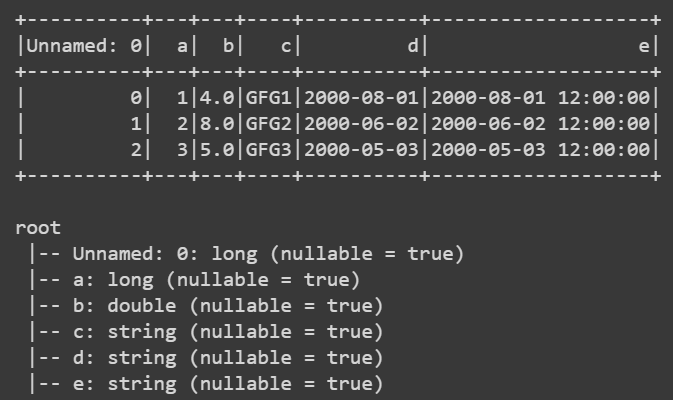### Create PySpark DataFrame from Text file

In the given implementation, we will create pyspark dataframe using a Text file. For this, we are opening the text file having values that are tab-separated added them to the dataframe object. After doing this, we will show the dataframe as well as the schema.

File Used: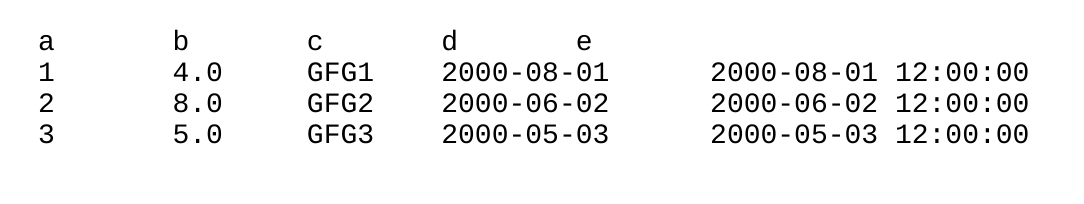## Python3

 `# Need to import to use date time``from` `datetime ``import` `datetime, date` `# need to import for working with pandas``import` `pandas as pd` `# need to import to use pyspark``from` `pyspark.sql ``import` `Row` `# need to import for session creation``from` `pyspark.sql ``import` `SparkSession` `# creating the session``spark ``=` `SparkSession.builder.getOrCreate()` `# PySpark DataFrame from a csv``df ``=` `spark.createDataFrame(pd.read_csv(``'data.txt'``, delimiter``=``"\t"``))``df` `# show table``df.show()` `# show schema``df.printSchema()`

Output:### Create PySpark DataFrame from JSON

In the given implementation, we will create pyspark dataframe using JSON. For this, we are opening the JSON file added them to the dataframe object. After doing this, we will show the dataframe as well as the schema.

JSON Used: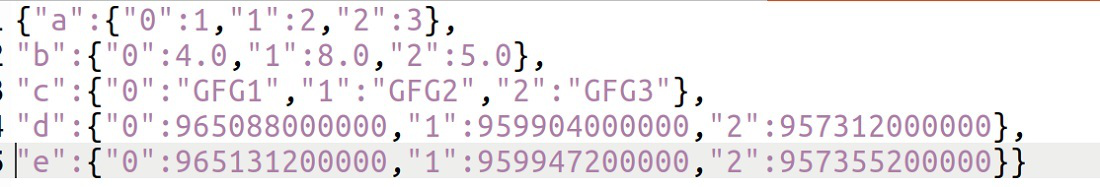## Python3

 `# Need to import to use date time``from` `datetime ``import` `datetime, date` `# need to import for working with pandas``import` `pandas as pd` `# need to import to use pyspark``from` `pyspark.sql ``import` `Row` `# need to import for session creation``from` `pyspark.sql ``import` `SparkSession` `# creating the session``spark ``=` `SparkSession.builder.getOrCreate()` `# PySpark DataFrame from a csv``df ``=` `spark.createDataFrame(pd.read_json(``'data.json'``))``df` `# show table``df.show()` `# show schema``df.printSchema()`

Output: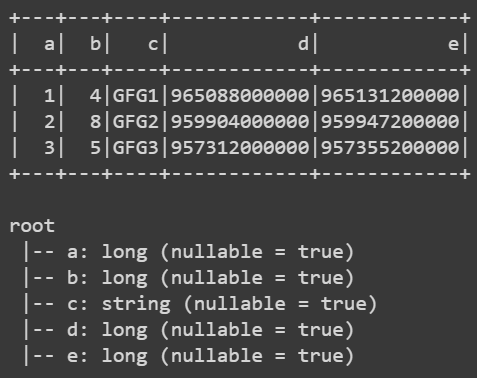So these all are the methods of Creating a PySpark DataFrame. The following datasets were used in the above programs.